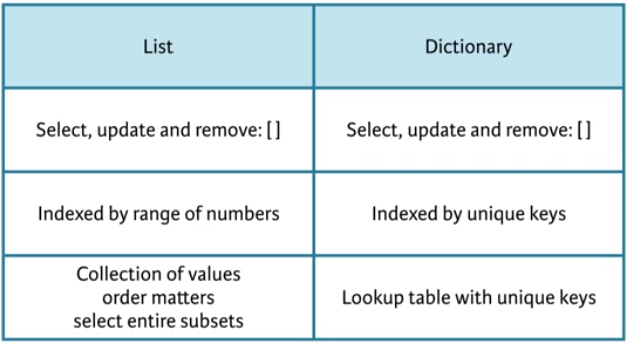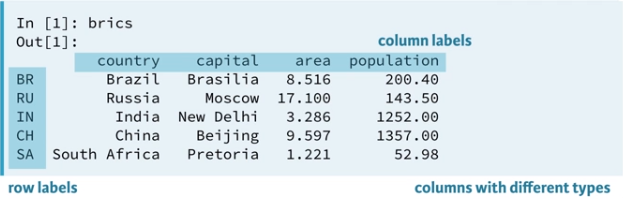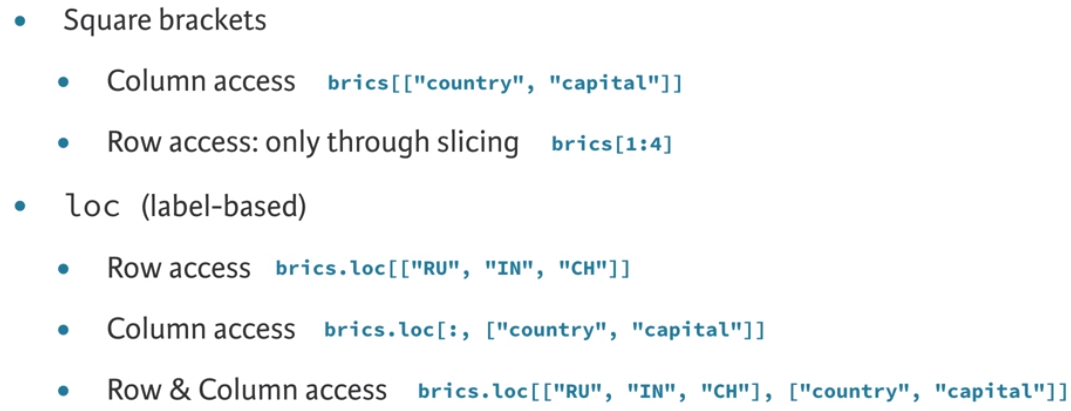# Intermediate Python for Data ScienceThe subjects in this DataCamp’s track, Intermediate Python for Data Science include:

• Matplotlib
• Dictionaries and Pandas
• Logic, Control Flow and Filtering
• Loops

It looks at data visualization – how to visualize data, data structures – how to store data. Along the way, it shows how control structures customize the flow of your scripts (codes).

### Data Visualization

It is one of the key skills for data scientists and Matplotlib makes it easy to create meaningful and informative charts. Matplotlib allows us to build various charts and customize them to make it more visually interpretable. It is not an hard thing to be done and it is pretty interesting to work on it. In my previous write-up, I wrote about how to use Matplotlib to build a line chart, scatter plot and histogram.

Data visualization is a very important part in data analysis. It helps to explore the dataset which it extracts insights. I call this as data profiling, the process of examine the dataset coming from existing data source such as databases, which consists of statistics or summaries of the dataset. The purpose is to find existing data can be used for other purposes, determine the accuracy, completeness and validity of the dataset. I can relate this to “perform a body check on the dataset to ensure it is healthy”.

One of the methods I learned from my school on data profiling is the use of histogram, scatter plot and boxplot to examine the dataset and find out the outliers. I can use either the Python’s Matplotlib, Excel, Power Bi or Tableau to perform this action.

### It does not end here…

Python allows us to do customization on the charts to suit our data. There are many types of charts and customization ones can do with Python, changing from colours, labels and axes’ tick size. It depends on the data and the story ones want to tell. Refer the links above to read my write-up on those charts.

### Dictionaries

We can use lists to store a collection of data and access the values using the indexes. It can be troublesome and inefficient when it comes to large dataset, therefore, the use of dictionaries in data analysis is important as it represents data in the form of key-value pairs. Creating a dictionary from the lists of data can be found in this link. It has one simple example demonstrating how to convert it. However, I do have a question, how about converting long lists to dictionary? I assumed it is not going to be the same method in this simple example. Does anyone have an example to share?

If you have questions about dictionaries, then you can refer to my blog which I wrote a quite comprehensive introduction of dictionaries in Python.

### What is the difference between lists and dictionaries?If you have a collection of values where order matters, and you want to easily select entire subsets, you will want to go with a list. On the other hand, if you need some sort of lookup table where looking for data should be fast, by specifying unique keys, dictionary is a preferred option.

### Lastly, Pandas

Pandas is a high level data manipulation tool built on top of NumPy package. Since NumPy 2D array allows to use one data type in their elements, it may not suitable for some of the data structure which comprise of more than one data type. In Pandas, data is stored like a tabular table called DataFrame, for example:### How to build a DataFrame?

There are few ways to build a Pandas DataFrame and we need to import Pandas package before we begin. In my blog, there are two methods shared, using dictionaries and external file such as .csv file. You can find the examples from the given link. Reading from dictionaries can be done by converting dictionary into DataFrame using DataFrame() and reading from the external file can be done using Pandas’ read_csv().

• Converting dictionary using DataFrame()

### How to read from a DataFrame?

The above screenshot shows how the Pandas’ DataFrame looks like, it is in the form of rows and columns. If you wonder why the first column goes without naming. Yes, in the .csv file it has no column name. It appears to be an identifier for each row, just like an index of the table or row label. I have no idea whether the content of the file is done with this purpose or it has other meaning.

### Index and Select Data

There are two methods you can select data:

• Using square bracket []
• Advanced methods: loc and iloc.

The advanced methods, loc and iloc is Python’s powerful, advanced data access. To access a column using the square bracket, with reference to the above screenshot again, the following codes demonstrate how to select that country column:

```brics["country"]
```

The result shows the row label together with the country column. This is how it read a DataFrame which it returns an object called Pandas Series, which you can assume Series is a one dimension labelled array and when a bunch of Series comes together then, it is called DataFrame.

If you want to do the same selection of country column and keep the data as DataFrame, then using the double square brackets, it can do the magic with following code:

```brics[["country"]]
```

If you check the type of the object, it returns as DataFrame. You can define more than one column to be returned. To access rows using the square bracket and slices, with reference to the same screenshot, the below code is used:

```brics[1:4]
```

The result returns the from row number 2 to 4 or index 1 to 3 which contains, Russia, India and China. If you still remember, the characteristic of slice? The stop value (end value) of a slice is exclusive (not included in the output.

However, this method is has a limitation. For example, if you want to access the data similar to 2D Numpy Array, it can be done using the square bracket with specific column and row.

```my_array[column, row]
```

Hence, Pandas has this powerful and advanced data access, loc and iloc, where loc is a label based and iloc is position based. Let us looking into the usage of loc. The first example reads row loc and follow by another example reads row and column loc. With the same concept as above, single square bracket returns a Series and double square brackets return a DataFrame, just as below:

```brics.loc["RU"] --Series single row
brics.loc[["RU"]] --DataFrame single row
brics.loc[["RU, "IN", "CH"]] --DataFrame multiple row
```

Let extends the above code to read country and capital columns using the row and column with loc. First part it mentions the rows and second part it mentions the column labels. The below code returns a DataFrame.

```brics.loc[["RU, "IN", "CH"], ["country", "capital"]]
```

The above rows values can be replaced with slice, just like the sample code below:

```brics.loc[:, ["country", "capital"]]
```

The above code did not specify the start and end index, it means it returns all the rows with country and capital columns. Below is the screenshot of comparison between square brackets and loc (label-based).Using iloc is similar to the loc, the only different is how you refer column and row which is using index instead of specifying the rows and column labels.